BBC Over Direct Sequence Spread Spectrum

(Last Mod: 27 November 2010 21:37:21 )

NOTE: WORK VERY MUCH IN PROGRESS

Introduction

Most of the examples that exist for the concurrent code based BBC encoding and decoding algorithms use pulse-based radios as the underlying physical layer. However, that is only one of the three dominant categories of spread spectrum with the others being frequency hopping and direct sequence. The application of BBC to frequency hopping is straightforward and won't be explored here, but whether BBC even can be applied to direct sequence spread spectrum (DS/SS) is not at all obvious. That's what this portion of the website is all about.

Overview of Direct Sequence Spread Spectrum

Direct sequence spread spectrum (DS/SS), which is at the heart of code division multiple access (CDMA) used in such applications as GPS and some cell phone networks, works by modulating an information signal with a pseudorandom bit stream. In essence there are two independent data sources, the information bit stream and the pseudorandom bit stream, that modulate each other. Just as a traditional narrowband receiver might lock onto a carrier and extracts the information that is modulating it, a DS/SS receiver locks on to the pseudorandom bit stream and extracts the information that is modulating it.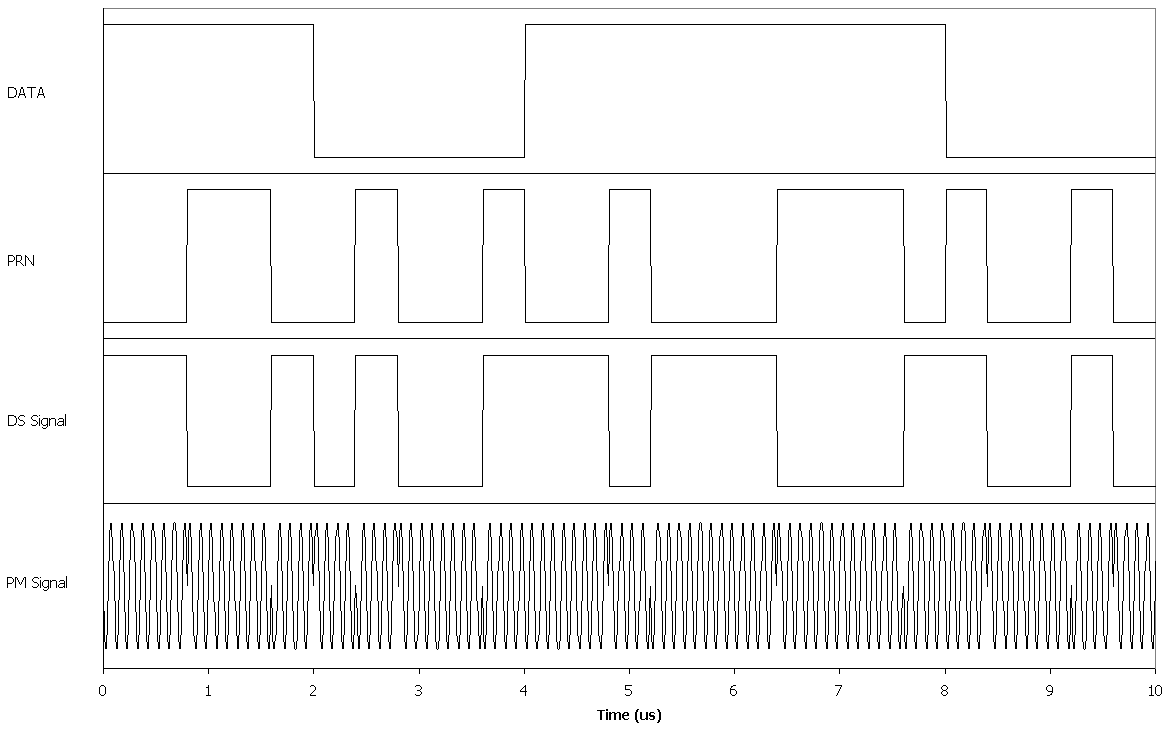GPS Gold Codes

The pseudorandom number (PRN) sequences used by GPS are not m-sequences, but are instead Gold code sequences. In general, Gold codes are generated by adding (modulo-2) two carefully chosen m-sequences. The result is a family of codes that have good cross-correlation properties at the expense of the very good autocorrelation properties exhibited by m-sequences.

For the purposes of this discussion, we will only consider the Gold codes used by the GPS C/A code. These codes are generated using the m-sequences produced by the following modulus polynomials:

By delaying one of the generators relative to the other, 1023 different Gold code sequences can be generated. The nominal hardware implementation of the GPS PRN generators can generate a subset consisting of 45 (possibly 55) of these. Of this set, 32 are assigned to satellites and an additional 5 are assigned to ground control stations although, for some reason, two of the ground station channels are assigned identical PRN sequences.

GPS Modulation

The GPS C/A signal carries data at 50 bps. The data is spread by that satellites Gold code at 1.023 chips/sec. Since the sequence is 1023 chips long, the sequence repeats every millisecond. The signal is modulated using Bi-Phase Shift Keying (BPSK) at a carrier frequency of 1575.42 MHz. The signal bandwidth is, roughly, 2 MHz and the nominal processing gain is approximately 20,460 (43 dB).

BBS over DSSS Simulation

To demonstrate the theoretical feasibility of of using traditional Direct Sequence Spread Spectrum as a substrate for a BBC-encoded channel, a simulation was performed in which a number of randomly chosen offsets of a pseudorandom number sequence were used. The sequences for all of these offsets were added and the resulting signal PSK modulated. The receiver then swept through the received signal performing a correlation for all possible offsets. The simulation was performed using an ANSI-C compliant program.

The following view graphs summarize the results.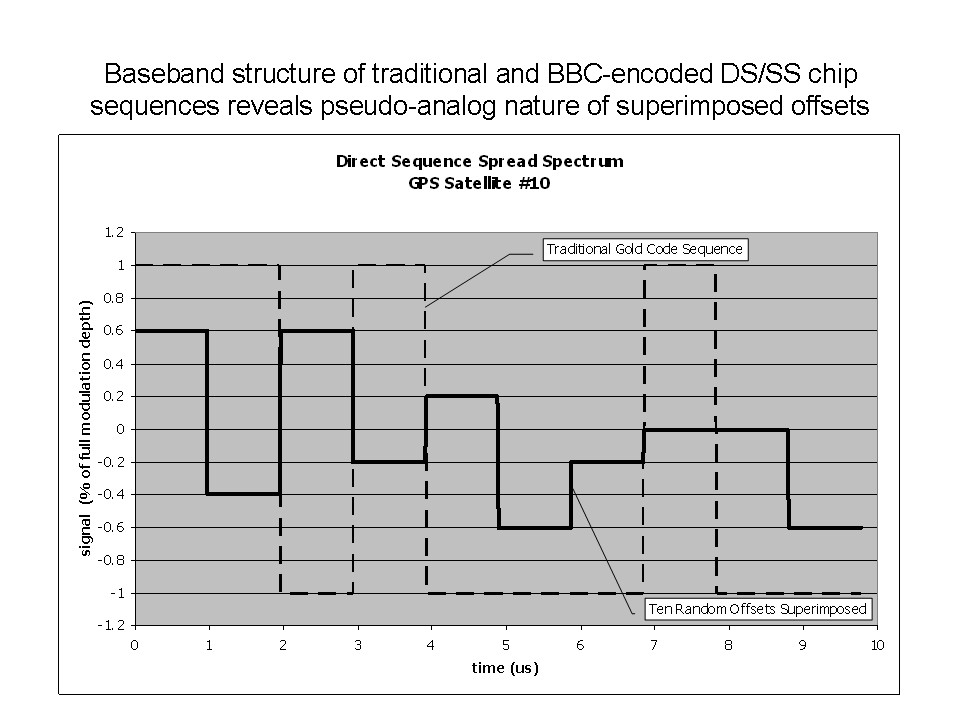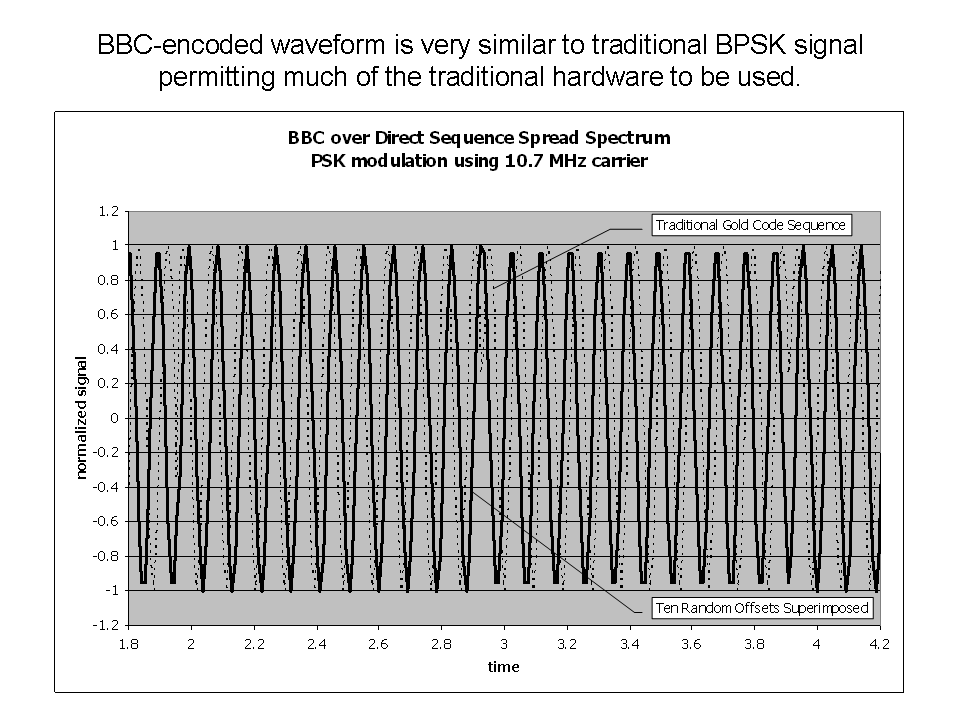The program included the ability to add white Gaussian noise to the signal at a specified power level. The following data was generated using a signal-to-noise ratio of 100dB. In other words, essentially noise-free. What this shows is the degree to which the transmitted offsets rise above the spurious levels in the non-transmitted offsets due to autocorrelation effects. Since one of the primary applications of this approach is GPS, the actual Gold Code pseudorandom sequence for GPS satellite #10 was used for these simulations. Thus the simulation takes into the account the relatively poor autocorrelation performance of the short Gold Codes actually in use by the GPS system.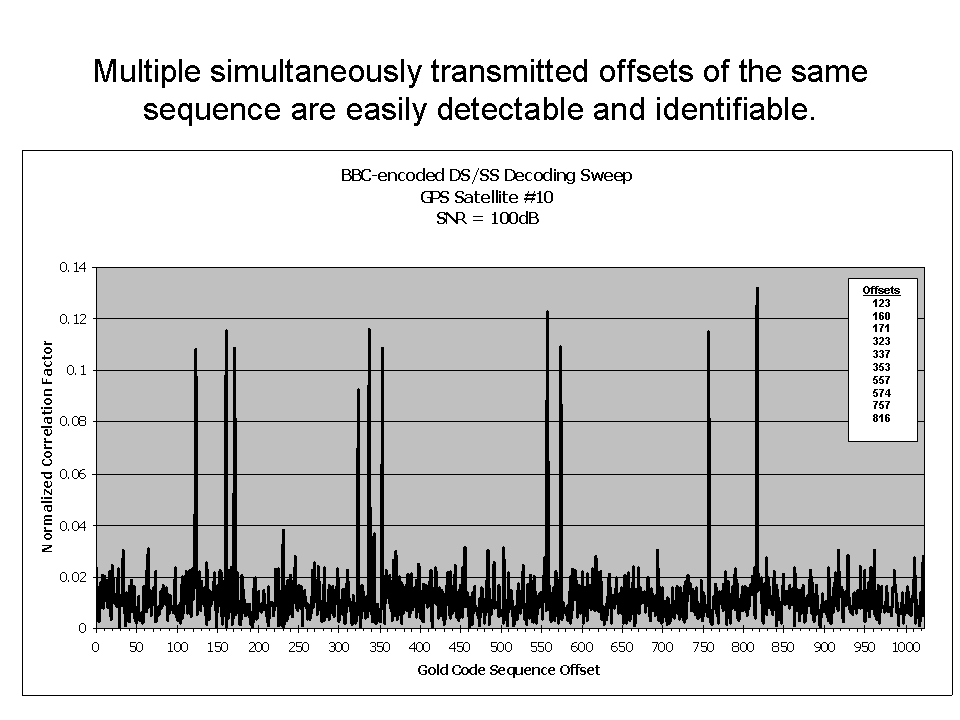To demonstrate how well the distinct offsets can be picked up in a signal buried in the noise, the following data is for a signal-to-noise ratio of -30dB, meaning that the noise has 1000 times the power of the total transmitted signal (which is spread across the ten offsets used). None-the-less, the offsets stand out clearly, although a couple of non-transmitted offsets have sufficient energy in them that they would be likely to recovered as marks by the discriminator, although recall that the BBC algorithms are specifically designed to be highly tolerant to false marks.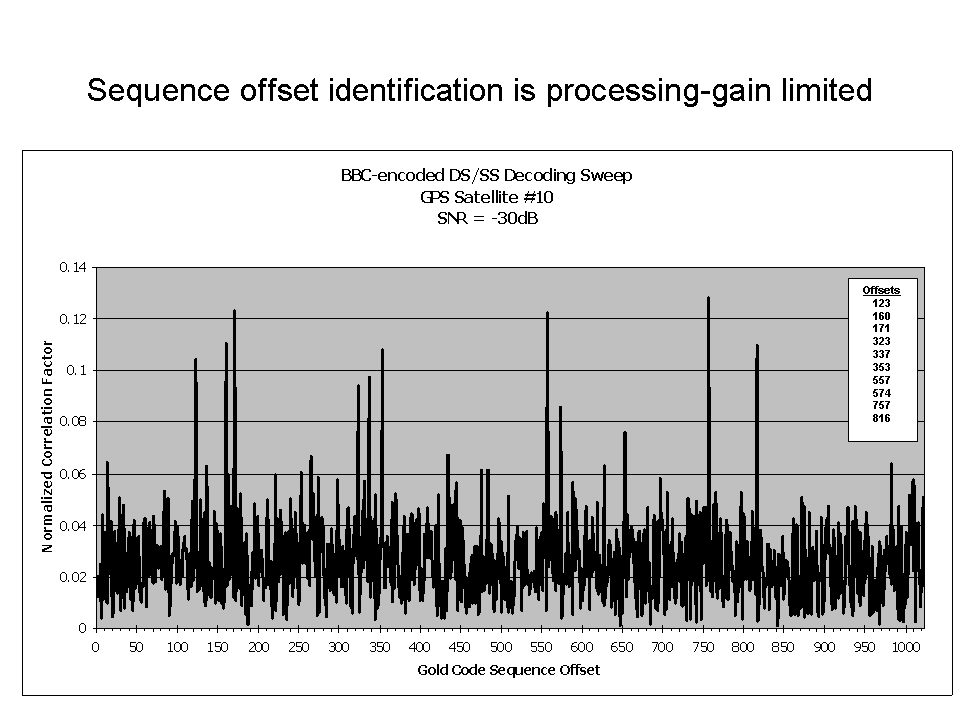Source Code

The following is the set of source code files needed to reproduce the above data. There is no claim at this point that the code is well documented.